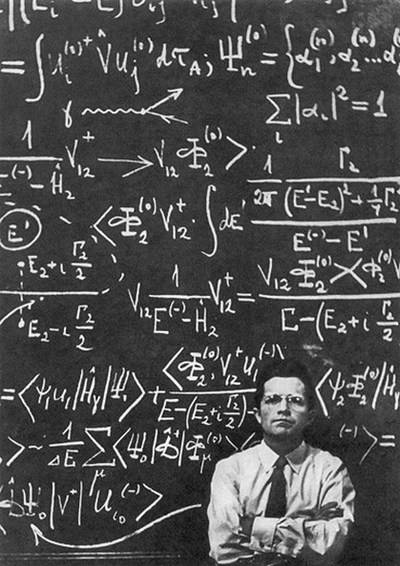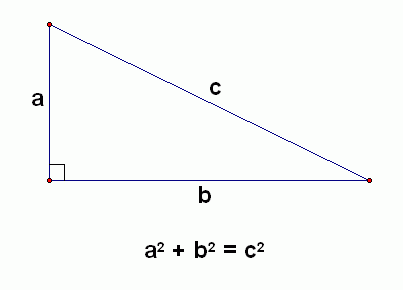# What was the most important mathematical equation ever discovered?

This is a great question!

I believe mathematics is the foundation of the world around us, so picking one equation that has proven to be "the most important" is tough.I guess the first equation that comes to mind is the Pythagorean theorem. It is the basis of trigonometry, and possibly the most important geometrical equation.

It looks like this:Why is it so important? Without it, we wouldn't have maps, geography, or GPS systems.

Navigating the oceans would have never happened without this theorem, and I'd hate to imagine where we'd be in modern times if we didn't know what was across the sea!

1 Person thanked the writer.

Another take on this, which isn't so much about our use of the formula, but instead about its very existence.

E=MC^2

The equation (The 'mass energy equivalence' equation), discovered by Albert Einstein, explains (amongst other things) the energy released in nuclear fusion and fission reactions like those that power our Sun.

If this formula didn't exist, there would be no life. Anywhere.

There would be no Earth, Mars, Venus, or Mercury since every element heavier than Beryllium was created in the nuclear furnaces of stars. The universe would be filled with billions upon billions of slowly cooling balls of hydrogen and helium.

I'm pretty thankful the equation holds.

On a lighter note, E=MC^2 also tells me (assuming I didn't screw up the math) that my body mass is enough to power the entire planet for a little over 49 seconds. I'll leave it as an excercise for the reader to determine if I need to lose weight...